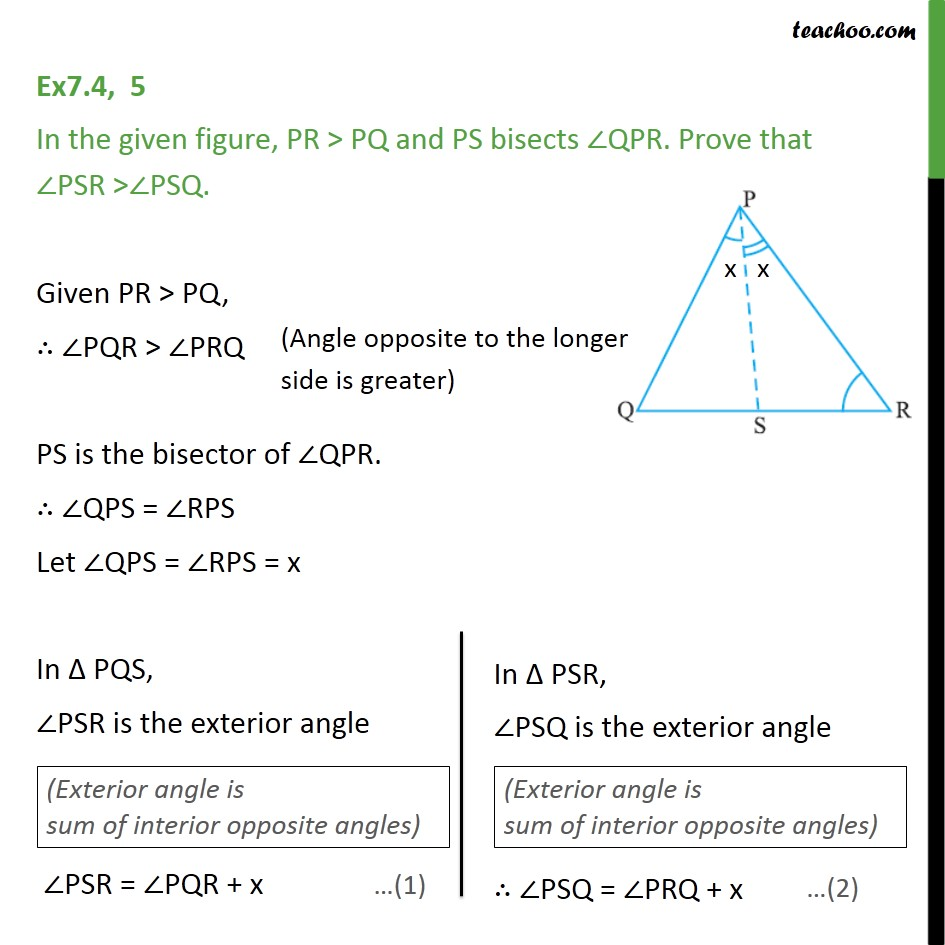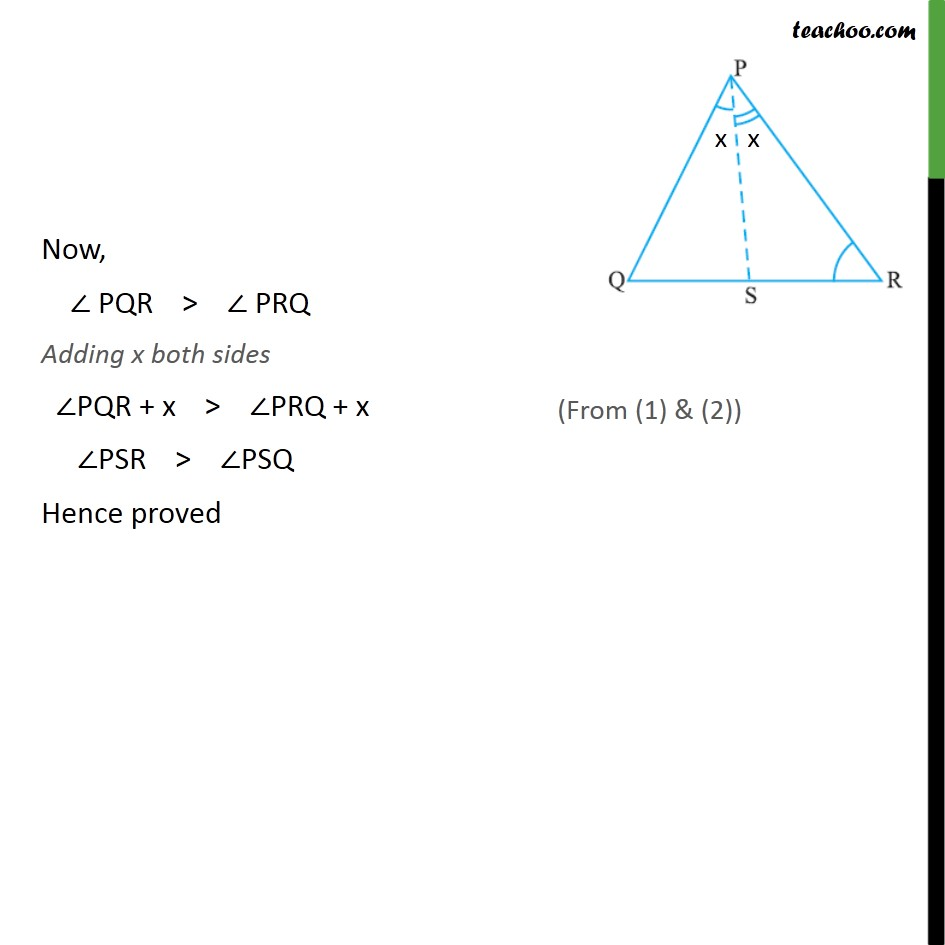1. Chapter 7 Class 9 Triangles
2. Serial order wise
3. Ex 7.4

Transcript

Ex7.4, 5 In the given figure, PR > PQ and PS bisects ∠QPR. Prove that ∠PSR >∠PSQ. Given PR > PQ, ∴ ∠PQR > ∠PRQ PS is the bisector of ∠QPR. ∴ ∠QPS = ∠RPS Let ∠QPS = ∠RPS = x In Δ PQS, ∠PSR is the exterior angle ∠PSR = ∠PQR + x Now, ∠ PQR > ∠ PRQ Adding x both sides ∠PQR + x > ∠PRQ + x ∠PSR > ∠PSQ Hence proved

Ex 7.4

Chapter 7 Class 9 Triangles
Serial order wise